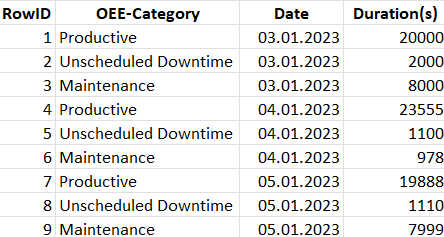# Calculate a Formula with multiple rows and condition

Hello everyone.
I am trying to calculate different OEE values of a machine using formulas.

My Data-Structure is as following (just an example, real data is more complex):To sum it up:

• The data is grouped by date and OEE-Category
• The Duration(s) Column shows how long each category was logged on that specific date

What I want to do
For example I would like to calculate the availability of the machine on each day. The Formula general formula therefore is:
Availability = actual production time / possible production time.

So if i want to calculate the machines availability on 03.01.2023 it would look like:
Availability = (57600 (2x8-Hour-Shifts in seconds) - 2000 (Unscheduled Downtime) - 8000 (Maintence)) / 57600 = 0,826 = 82,6%

I already tried several approaches but I really don’t know how to do such an operation with knime-nodes.